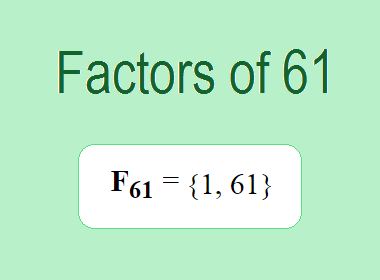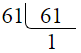# Factors of 61The factors of 61 are 1 and 61 i.e. F61 = {1, 61}. The factors of 61 are all the numbers that can divide 61 without leaving a remainder. 61 is a prime number, so it is divisible by 1 and 61 only.

We can check if these numbers are factors of 61 by dividing 61 by each of them. If the result is a whole number, then the number is a factor of 61. Let's do this for each of the numbers listed above:

·        1 is a factor of 61 because 61 divided by 1 is 61.

·        61 is a factor of 61 because 61 divided by 61 is 1.

## Properties of the Factors of 61

The factors of 61 have some interesting properties. One of the properties is that the sum of the factors of 61 is equal to 62. We can see this by adding all the factors of 61 together:

1 + 61 = 62

Another property of the factors of 61 is that the only prime factor of 61 is 61 itself.

********************

********************

## Applications of the Factors of 61

The factors of 61 have several applications in mathematics. One of the applications is in finding the highest common factor (HCF) of two or more numbers. The HCF is the largest factor that two or more numbers have in common. For example, to find the HCF of 61 and 122, we need to find the factors of both numbers and identify the largest factor they have in common. The factors of 61 are 1, and 61. The factors of 122 are 1, 2, 61, and 122. The largest factor that they have in common is 61. Therefore, the HCF of 61 and 122 is 61.

Another application of the factors of 61 is in prime factorization. Prime factorization is the process of expressing a number as the product of its prime factors. The prime factor of 61 is 61 since it is only the prime number that can divide 61 without leaving a remainder. Therefore, we can express 61 as:

61 = 61

We can do prime factorization by division method as given below,61 = 61

Since 61 is a prime number, there is no factor tree of 61.

## Conclusion

The factors of 61 are the numbers that can divide 61 without leaving a remainder. The factors of 61 are 1, and 61. The factors of 61 have some interesting properties, such as having a sum of 62. The factors of 61 have several applications in mathematics, such as finding the highest common factor and prime factorization.